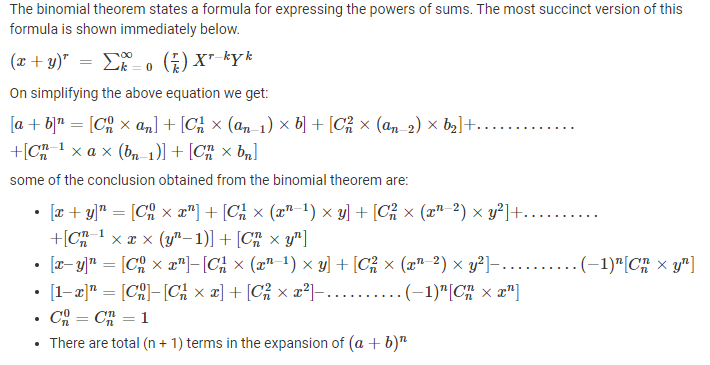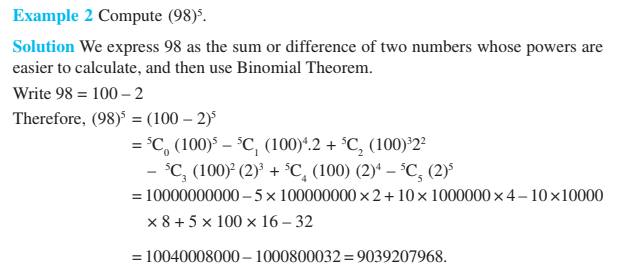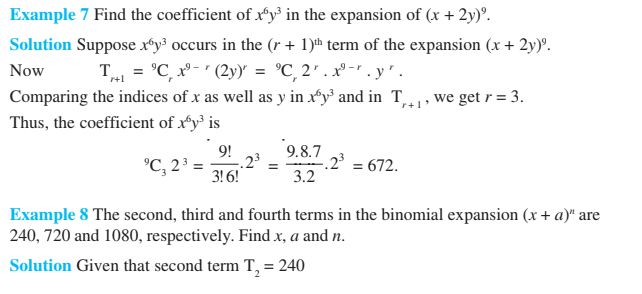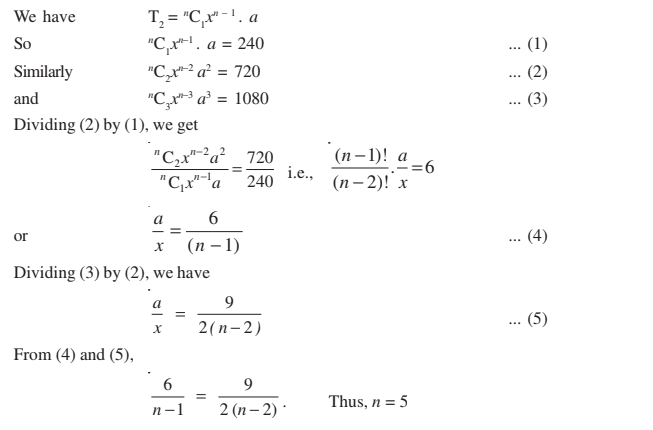# Binomial Theorem Class 11

In binomial theorem class 11, chapter 8 provides the information regarding the introduction and basic definitions for binomial theorem in a detailed way. To score good marks in binomial theorem class 11 concepts, go through the given problems here. Solve all class 11 Maths chapter 8 problems in the book by referring the examples to clear your concepts on binomial theorem.

## Binomial Theorem Class 11 Topics

The topics and sub-topics covered in binomial theorem class 11 are:

• Introduction
• Binomial theorem for positive integral indices
• Binomial theorem for any positive integer n
• Special Cases
• General and Middle Term

## Binomial Theorem Class 11 Notes### Binomial Theorem Class 11 ExamplesStay tuned with BYJU’S – The Learning App to gain knowledge on binomial theorem concepts and also refer more practice problem to score more problems.